Lambert W function (for HP Prime)
10-25-2020, 08:31 AM (This post was last modified: 11-23-2020 06:39 AM by lyuka.)
Post: #1
 lyukaJunior Member Posts: 48 Joined: May 2014
Lambert W function (for HP Prime)
Hi guys,
a few days ago my wife bought me an HP Prime (2AP18AA) as a little early birthday present.
I'm completely new to HP Prime, but I implemented the Lambert W function and the expornent of the Lambert W function that I wrote the other day as a trial to get started.

Please refer to the page below for details.
Lambert W function (for HP Prime)

Code:
 //W0 - Principal branch of the Lambert W function //Rev.1.1 (Oct. 25, 2020) (c) Takayuki HOSODA (aka Lyuka) EXPORT W0(x) BEGIN   LOCAL y, r, t, i;   r := 1 / e;   IF (x == -r) THEN     return -1;   ELSE     t := x + r;     y := ln(r + sqrt((r + r) * t) + 0.3040682660859502 * t); // approximation near x=-1/e     FOR i FROM 0 TO 16 DO       r := t;       t := exp(y);       IF (-1 == y) THEN break; END;       t := (x - y * t) / (t * (1.0 + y)); // Newton-Raphson method       y := y + t;       IF (abs(t) >= abs(r) AND i > 0) THEN break; END; // convergence check     END;   END;   return y; END;

Code:
 //eW : exponent of the Lambert W0 function //Rev.1.1 (Oct. 25, 2020) (c) Takayuki HOSODA (aka Lyuka) EXPORT eW(x) BEGIN   LOCAL y, r, t, u, v, i;   r := 1 / e;   IF (x == -r) THEN     return r;   ELSE     t := x + r;     y := r + sqrt((r + r) * t) + 0.3040682660859502 * t; // approximation near x=-1/e     FOR i FROM 0 to 10 DO       r := t;       t := ln(y);       v := x - y * t;       t := t + 1;       u := (t + t) * y;       t := v + u * t;       IF (0 == t) THEN break; END;       t := u * v / t; // Halley's method       y := y + t;       IF (abs(t) >= abs(r) AND i > 0) THEN break; END; // convergence check     END;   END;   return y; END;
10-25-2020, 07:02 PM
Post: #2
 Albert ChanSenior Member Posts: 2,357 Joined: Jul 2018
RE: Lambert W function (for HP Prime)
Does HP Prime have LambertW built-in ?

XCas> solve(x^2 = 2^x, x)

[-exp(-LambertW(ln(2)/2)), exp(-LambertW(-ln(2)/2)), exp(-LambertW(-ln(2)/2,-1))]

XCas> float(ans())

[-0.766664695962, 2.0, 4.0]

---

I think it is safe to use Halley's method for W.
Think of Halley's method as correction to Newton's method slope.
For W, slope correction is at most 50% (when x is *far* from -1/e)
10-27-2020, 03:43 AM (This post was last modified: 10-30-2020 04:55 AM by lyuka.)
Post: #3
 lyukaJunior Member Posts: 48 Joined: May 2014
RE: Lambert W function (for HP Prime)
(10-25-2020 07:02 PM)Albert Chan Wrote:  Does HP Prime have LambertW built-in ?

XCas> solve(x^2 = 2^x, x)

[-exp(-LambertW(ln(2)/2)), exp(-LambertW(-ln(2)/2)), exp(-LambertW(-ln(2)/2,-1))]

XCas> float(ans())

[-0.766664695962, 2.0, 4.0]

---

I think it is safe to use Halley's method for W.
Think of Halley's method as correction to Newton's method slope.
For W, slope correction is at most 50% (when x is *far* from -1/e)
HP Prime doesn't seem to have LambertW function. I found a previous thread on this.
Xcas/Giac vs HP Prime: LambertW

--
I reconfirmed the Halley method.
The precision of the least significant digit is often better than Newton's method,
and I haven't encountered any unstable behavior so far,
so I changed the program to use Halley's method.
The program name has been changed from W0 to LW0.
Code:
 //LW0 - Principal branch of the Lambert W function //Rev.1.22 (Oct. 30, 2020) (c) Takayuki HOSODA (aka Lyuka) EXPORT LW0(x) BEGIN   LOCAL y, r, s, t, i;   r := 1 / e;   IF (x == -r) THEN return -1; END;   t := x + r;   y := ln(r + sqrt((r + r) * t) + 0.3040682660859502 * t); // approximation near x=-1/e   FOR i FROM 0 TO 10 DO     r := t;     t := exp(y);     s := y * t;     t := (1 + 0.5 * y) * (s + x) + t;     IF (t == 0) THEN break; END;     t := (y + 1) * (s - x) / t; // Halley's method     y := y - t;     IF (t == 0 OR abs(t) >= abs(r) AND i > 0) THEN break; END; // convergence check   END;   return y; END;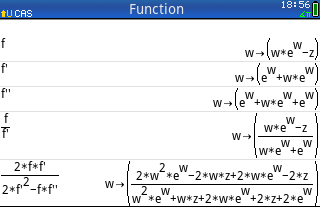Please refer to the page below for details.
Lambert W function and exponent of Lambert W function for HP Prime
10-27-2020, 04:01 PM
Post: #4
 Albert ChanSenior Member Posts: 2,357 Joined: Jul 2018
RE: Lambert W function (for HP Prime)
(10-27-2020 03:43 AM)lyuka Wrote:  I reconfirmed the Halley method.
The precision of the least significant digit is often better than Newton's method,
and I haven't encountered any unstable behavior so far,
so I changed the program to use Halley's method.

Good call.
For W, Halley's method is safer than Newton's method. (safest is e^W approach)

Newton's method: x ← x - N, where N = f/f'
For W(a), we setup f = x*e^x - a, f' = x*e^x + e^x

XCas> N := (x*e^x - a) / (x*e^x + e^x)
XCas> limit(N, x=+inf)﻿ ﻿ ﻿ → 1
XCas> limit(N, x=-inf)﻿ ﻿ ﻿ ﻿ → inf
XCas> limit(N, x=-1)﻿ ﻿ ﻿ ﻿ ﻿ ﻿ → inf

Halley's method: x ← x - H, ﻿ ﻿ where H = f / (f' - (f/f')/2*f'') = N / (1 - N/2*(f''/f'))
f'' = (f')' = (f + e^x - a)' = f' + e^x
f''/f' = 1 + e^x/f' = 1 + 1/(1+x)

XCas> H := N/(1 - N/2*(1+1/(1+x)))
XCas> limit(H, x=+inf)﻿ ﻿ ﻿ → 2
XCas> limit(H, x=-inf)﻿ ﻿ ﻿ ﻿ → -2
XCas> limit(H, x=-1)﻿ ﻿ ﻿ ﻿ ﻿ ﻿ → 0

With bad guess, Newton's correction close to singularity may hugely overshoot.
And, it does not take much to have a bad guess, see the tests below ...

Code:
from mpmath import * r, c = 1/e, e-sqrt(2)-1 def w_newton_corr(x, a):     ex = exp(x)     return (x*ex - a) / (x*ex + ex) w_guess = lambda x,c=c: log(sqrt(2*r*(x+r)) + c*(x+r) + r) w_newton = lambda x, a: x - w_newton_corr(x,a) w_halley = lambda x, a: x - 1/(1/w_newton_corr(x,a) - (.5+.5/(1+x)))

>>> def nest(func, x, a, n=6):
... ﻿ ﻿ ﻿ ﻿ ﻿ ﻿ ﻿ ﻿ ﻿ ﻿for i in range(n): print i,x; x = func(x,a)

>>> x = -1e99
>>> w = 200+3j # bad guess, but only 10% off, W(x) = 222.550670661503 + 3.12754041755172j

>>> nest(w_newton , w, x) # Newton totally failed
0 (200+3j)
1 (6829135788.03541 + 869691954.442808j)
2 (6829135787.03541 + 869691954.442808j)
3 (6829135786.03541 + 869691954.442808j)
4 (6829135785.03541 + 869691954.442808j)
5 (6829135784.03541 + 869691954.442808j)

>>> nest(w_halley, w, x) # 14 iterations to converge
0 (200+3j)
1 (201.990101192677 + 3.00014701205407j)
2 (203.98029891143 + 3.00029117778268j)
3 (205.970591273429 + 3.00043258190625j)
4 (207.960976300103 + 3.00057132498455j)
5 (209.951450963988 + 3.00070764455423j)

Same behavior (but no complex numbers) if we try x = 1e99, guess w = 200.

---

>>> x = -1/e + 1e-5 # close to singularity
>>> w = -1 + 1e-10 # guess too close to singularity, W(x) = -0.992644755197123

>>> nest(w_newton, w, x) # 272,000 iterations to converge
0 -0.9999999999
1 271827.05391625
2 271826.053919929
3 271825.053923608
4 271824.053927287
5 271823.053930966

>>> nest(w_halley, w, x) # 19 iterations to converge
0 -0.9999999999
1 -0.9999999997
2 -0.9999999991
3 -0.9999999973
4 -0.999999991899999
5 -0.999999975699998

With good guess, w_guess(x) = -0.992645539358962, we don't have above problems.
w_newton will converge in 2 iterations, w_halley in 1.
10-30-2020, 12:19 AM
Post: #5
 Albert ChanSenior Member Posts: 2,357 Joined: Jul 2018
RE: Lambert W function (for HP Prime)
(10-27-2020 04:01 PM)Albert Chan Wrote:  For W, Halley's method is safer than Newton's method. (safest is e^W approach)

We can get the safety of e^W approach, without recovering W at the end.
Since w_guess(0) = W(0), we can remove the case for W(a = 0):

x * exp(x) = a, ﻿ ﻿ ﻿ ﻿ ﻿ ﻿ a ≠ 0
ln(x) + x = ln(a)

f = x + ln(x/a) = 0
f' = 1 + 1/x = (x+1)/x
f'' = -1/x^2
f''/f' = -1/(x*x+x)

This is version 2, using above f setup:
Code:
w_newton2_corr = lambda x, a: (x + log(x/a)) * x/(x+1) w_newton2 = lambda x, a: x - w_newton2_corr(x,a) w_halley2 = lambda x, a: x - 1/(1/w_newton2_corr(x,a) + .5/(x*x+x)) def nest(func, x, a, n=6):     for i in range(n):         print i,x;         try: x = func(x,a)         except ZeroDivisionError: break

Now, redo the bad guess example, and see the improvements:

>>> from mpmath import *
>>> x = -1e99
>>> w = 200 + 3j # bad guess

>>> nest(w_newton2, w, x)
0 (200+3j)
1 (222.54478620735 + 3.12764616976661j)
2 (222.550670661156 + 3.12754041757397j)
3 (222.550670661503 + 3.12754041755172j) <-- converged in 3
4 (222.550670661503 + 3.12754041755172j)
5 (222.550670661503 + 3.12754041755172j)

>>> nest(w_halley2, w, x)
0 (200+3j)
1 (222.551107406185 + 3.12752854221077j)
2 (222.550670661503 + 3.12754041755172j) <-- converged in 2
3 (222.550670661503 + 3.12754041755172j)
11-02-2020, 09:53 AM (This post was last modified: 11-03-2020 05:48 AM by lyuka.)
Post: #6
 lyukaJunior Member Posts: 48 Joined: May 2014
RE: Lambert W function (for HP Prime)
Whether it's practical or not, I was interested in higher-order convergence methods,
so I wrote a function to calculate LambertW using Householder's method of order 4.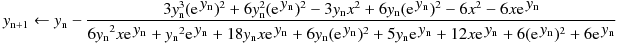The convergence test by Urabe's theorem is used to determine the convergence.
It is a method to judge that the calculation limit has been reached and terminate
the iteration when the correction value does not change or increases.

Code:
 //LW0H - Principal branch of the Lambert W function //Rev.1.34 (Nov. 2, 2020) (c) Takayuki HOSODA (aka Lyuka) EXPORT LW0H(x)   LOCAL y, p, r, s, t, u, v;   HComplex := 1; // Enable complex result from a real input.   r := 1 / e;   IF (x == -r) THEN return -1; END;   s := x + r;   y := ln(r + sqrt((r + r) * s) + 0.3040682660859502 * s); // approximation near x=-1/e   t := 0;   REPEAT     p := y;     r := t;     s := exp(y);     u := y * s;     v := y + 2;     t := s + s;     t := 3 * (u * (u * v + t) - x * (x * v + t));     v := (y + 3) * ( u * u + x * (x + 4 * u)) + 6 * s * (u + x + x + s);     IF (0 == v) THEN break; END;     t := t / v;     y := y - t; // Householder's method of order 4     t := abs(t);   UNTIL p == y OR 0 == t OR (t >= r AND 0 <> r); // convergence check by Urabe's theorem   return y; END;

Example of fourth-order convergence
LW0H(3) → 1.04990889496403995998869707...
#iteration, t, y
0, -- , 1.08724606477
1, 3.73371212519e-2 , 1.04990894352
2, 4.85551153205e-8 , 1.04990889496
3, -4.97651234081e-12, 1.04990889496

Example of convergence check by Urabe's method
LW0H(-1e40+1e40*i) → 87.9726013585729060233240600422... + 2.32971836088312317016079031477...*i
#Iteration, t, y
0, y = 91.2594742667+2.35619449019*i
1, t = 2.58701135424 +9.47329698771e-3 *i, y = 88.6724629125+2.3467211932 *i
2, t = 0.698946120062 +1.68949509172e-2 *i, y = 87.9735167924+2.32982624228*i
3, t = 9.15433828888e-4 +1.07881392113e-4 *i, y = 87.9726013586+2.32971836089*i
4, t = 2.77222531883e-11+9.83926571672e-12*i, y = 87.9726013586+2.32971836088*i
5, t = 2.45892275992e-11-5.24426278827e-12*i, y = 87.9726013586+2.32971836089*i
6, t = 2.77222531883e-11+9.83926571672e-12*i, y = 87.9726013586+2.32971836088*i

In this case, the convergence test (|t6| > |t5|) by Urabe's method worked well.

Please refer to the page below for details.
Lambert W function and exponent of Lambert W function for HP Prime

P.S.
A typo (by copy-paste-edit) was corrected, the code LW0H-1.34.hppgrm on the site above is OK.
11: 0 :- t; → t := 0;
11-02-2020, 06:37 PM (This post was last modified: 11-03-2020 02:07 PM by Albert Chan.)
Post: #7
 Albert ChanSenior Member Posts: 2,357 Joined: Jul 2018
RE: Lambert W function (for HP Prime)
(11-02-2020 09:53 AM)lyuka Wrote:  The convergence test by Urabe's theorem is used to determine the convergence.
It is a method to judge that the calculation limit has been reached and terminate
the iteration when the correction value does not change or increases.

We are assuming guess is good enough that we never get a false positive.
With your guess function, I think we are safe, but hard to be sure.

Example, if we try iterating for W(1e99), guess = 200, we get this:
Code:
n  O(4) correction   W(1e99) 1  2.98522167315105  202.985221673151   2  2.98543581371237  205.970657486863   3  2.98564322111156  208.956300707975   4  2.98583215049592  211.942132858471   5  2.98576178491479  214.927894643386   6  2.98060044381505  217.908495087201   7  2.87730531320572  220.785800400406   8  1.69794037402345  222.483740774430   9  0.06702817368893  222.550768948119   10 0.00000000763134  222.550768955750 <-- converged

Supplied guess is 10% low. "Chaos" did not stop until 5th iteration.
Trivia: 4-th order correction limited to ±3, similar to 3-rd order Halley ±2

It is better if we apply convergence test after we reached half-precision.
Actually, half-precision test alone is enough here, since next iteration does nothing.

Even better, flatten the curve (see my previous post)
Newton's method beat Householder's method of order 4, converged in 3 iterations.

>>> nest(w_newton2, 200, 1e99)
0 200
1 222.544882427724
2 222.550768955402
3 222.55076895575
4 222.55076895575
5 222.55076895575

---

A few suggestions to LW0H(x) code

1) 0 := t may be wrong, should be t := 0

Better yet, t := 9, with a more robust test:

< UNTIL p == y OR 0 == t OR (t >= r AND 0 <> r);
> UNTIL p == y OR (r < 1 AND t >= r);

Since r < 1, we are in the safe zone.
Convergence test will not generate false positives, even with bad guess.

I removed 0 == t, since p == y already covered it.

2) if we detected chaos, updated iteration likely worse than before.

< return y;
> return p;

3) correction numerator can be made more accurate

< v := y + 2;
< t := s + s;
< t := 3 * (u * (u * v + t) - x * (x * v + t));

We expected u = y*exp(y) ≈ x, thus u-x is exact

> t := 3 * (u-x) * ((u+x)*(y+2) + 2*s)

Update:

4) correction denominator can be made more accurate, as polynomial of (u-x)

< v := (y + 3) * ( u * u + x * (x + 4 * u)) + 6 * s * (u + x + x + s)
> v := 6*((y+1)*u*x + (s+x)*(s+x+u)) + (u-x) * (3*(u+x) + y*(u-x))

The constant term is written this way because around singularity x≈-1/e, y≈-1, s≈-x
11-04-2020, 08:12 PM
Post: #8
 lyukaJunior Member Posts: 48 Joined: May 2014
RE: Lambert W function (for HP Prime)
Hi Albert.
Thank you for your informative suggestions.

(11-02-2020 06:37 PM)Albert Chan Wrote:  A few suggestions to LW0H(x) code

1) 0 := t may be wrong, should be t := 0

Better yet, t := 9, with a more robust test:

< UNTIL p == y OR 0 == t OR (t >= r AND 0 <> r);
> UNTIL p == y OR (r < 1 AND t >= r);

Since r < 1, we are in the safe zone.
Convergence test will not generate false positives, even with bad guess.

I removed 0 == t, since p == y already covered it.

I understand and agree with your early convergence test concept.

(11-02-2020 06:37 PM)Albert Chan Wrote:  2) if we detected chaos, updated iteration likely worse than before.

< return y;
> return p;

"likely worse than before" This may or may not be true under chaotic behavior, so I'm not sure whether to return y or p. I would like to return y so far.

(11-02-2020 06:37 PM)Albert Chan Wrote:  3) correction numerator can be made more accurate

< v := y + 2;
< t := s + s;
< t := 3 * (u * (u * v + t) - x * (x * v + t));

We expected u = y*exp(y) ≈ x, thus u-x is exact

Thank you very much. I understand and confirm that your formula has less error.

(11-02-2020 06:37 PM)Albert Chan Wrote:  > t := 3 * (u-x) * ((u+x)*(y+2) + 2*s)

Update:

4) correction denominator can be made more accurate, as polynomial of (u-x)

< v := (y + 3) * ( u * u + x * (x + 4 * u)) + 6 * s * (u + x + x + s)
> v := 6*((y+1)*u*x + (s+x)*(s+x+u)) + (u-x) * (3*(u+x) + y*(u-x))

The constant term is written this way because around singularity x≈-1/e, y≈-1, s≈-x

I understand and confirm that your denominator formula also has less calculation error.
However, the order of addition in the (s + x + u) term is also important, and when the order is ((s + x) + u), that is, ((e^y + x) + y * e^y) In the case of order, the calculation error due to digit loss seems to be reduced.

Code updated.
Code:
//LW0H - Principal branch of the Lambert W function //Rev.1.36 (Nov. 5, 2020) (c) Takayuki HOSODA (aka Lyuka) EXPORT LW0H(x) BEGIN   LOCAL y, p, r, s, t, u, v;   HComplex := 1; // Enable complex result from a real input.   r := 1 / e;   IF (x == -r) THEN return -1; END;   s := x + r;   t := e - sqrt(2) - 1;   y := ln(r + sqrt((r + r) * s) + t * s); // approximation near x=-1/e   t := 9;   REPEAT     p := y;     r := t;     s := exp(y);     u := y * s;     v := u - x;     t := s + x;     v := 6 * ((y + 1) * u * x + t * (t + u)) + v * (3 * (u + x) + y * v);     IF (0 == v) THEN break; END;     t := 3 * (u - x) * ((u + x) * (y + 2) + s + s);     t := t / v;     y := y - t; // Householder's method of order 4     t := abs(t);   UNTIL p == y OR 0 == t OR (r < 1 AND r <= t); // convergence check   return y; END;

By the way, in extreme cases such as x = -1.78, iterative calculation of about 16 times is required for any of Householder method of order 4, Halley's method, and Newton-Raphson method to converge. In this case, the state of convergence is not chaotic but very slowly and monotonously.

LW0H(-1.78)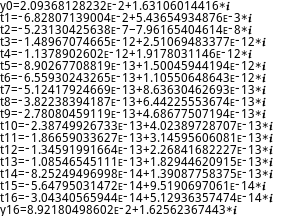lambertw(-1.78) by WolframAlpha is,
0.089218049856209317716109060765294251305387407793167833503833064... +
1.6256236744277681331520891017303249073577948841857860157186500... i

As a convergence decision in such a case, I wonder whether I should wait for convergence or stop the calculation when the appropriate accuracy is reached.
Though it depends, it seems too much to do 16 iterations for a calculation result of less than 12 digits.

I'm new to HP Prime so I'm not very familiar with it.
Can HP Prime calculate with arbitrary precision?
11-05-2020, 12:38 AM
Post: #9
 Albert ChanSenior Member Posts: 2,357 Joined: Jul 2018
RE: Lambert W function (for HP Prime)
(11-04-2020 08:12 PM)lyuka Wrote:
(11-02-2020 06:37 PM)Albert Chan Wrote:  2) if we detected chaos, updated iteration likely worse than before.

< return y;
> return p;

"likely worse than before" This may or may not be true under chaotic behavior, so I'm not sure whether to return y or p. I would like to return y so far.

I should re-phrase it.
It may slightly improve the iteration; Or, it can make it much worse.
"Chaos" test only gives the lower bound of how chaos the correction is.

Previous iteration is the safer choice.

Quote:By the way, in extreme cases such as x = -1.78, iterative calculation of about 16 times is required for any of Householder method of order 4, Halley's method, and Newton-Raphson method to converge. In this case, the state of convergence is not chaotic but very slowly and monotonously.

This has less to do with the algorithms, and more to do with HP Prime.
CAS side only have 48-bits precision (bottom 5 bits used internally).
Worse, rounding is *biased*, toward zero.

CAS> |(4./3-1)*3-1| ﻿ ﻿ ﻿ ﻿ ﻿ ﻿ → 1.42108547152e−14 = 2^-46

IEEE double of 4/3 = 0x1.5555555555555
Chop 5 bits, we have 0x1.555555555554

lua> abs((0x1.555555555554 - 1) * 3 - 1) ﻿ ﻿ ﻿ ﻿ ﻿ ﻿ → 1.4210854715202004e-014

I tried Householder O(4) code, it work as expected. (not 1 sided slow convergence)
Code:
def w_householder4(y, x):     s = exp(y)     u = y*s     t = 3 * (u-x) * ((u+x)*(y+2) + 2*s)     v = 6*((y+1)*u*x + (s+x)*(s+x+u)) + (u-x)*(3*(u+x) + (u-x)*y)     return y - t/v

>>> a = -1.78
>>> nest(w_newton, w_guess(a), a)
0 (0.0209368128240736 + 1.63106014415716j)
1 (0.0920804516694622 + 1.62406253942917j)
2 (0.0892198580409557 + 1.62561672078216j)
3 (0.0892180498219171 + 1.62562367442119j)
4 (0.0892180498562093 + 1.62562367442777j)
5 (0.0892180498562094 + 1.62562367442777j)
>>> nest(w_halley, w_guess(a), a)
0 (0.0209368128240736 + 1.63106014415716j)
1 (0.0891964913881074 + 1.62567299425531j)
2 (0.0892180498562183 + 1.62562367442774j)
3 (0.0892180498562093 + 1.62562367442777j)
4 (0.0892180498562094 + 1.62562367442777j)
5 (0.0892180498562093 + 1.62562367442777j)
>>> nest(w_householder4, w_guess(a), a)
0 (0.0209368128240736 + 1.63106014415716j)
1 (0.0892175267231459 + 1.62562359481102j)
2 (0.0892180498562093 + 1.62562367442777j)
3 (0.0892180498562094 + 1.62562367442777j)
4 (0.0892180498562093 + 1.62562367442777j)
5 (0.0892180498562094 + 1.62562367442777j)

Quote:Can HP Prime calculate with arbitrary precision?

Not for float.
But, you can use fsolve

CAS> a := -1.78
CAS> g := 2.09368128241e−2 + 1.63106014416*i
CAS> fsolve(x + ln(x/a) = 0, x = g)

8.92180498562e−2 + 1.62562367443*i
11-05-2020, 03:18 PM (This post was last modified: 11-05-2020 08:24 PM by Albert Chan.)
Post: #10
 Albert ChanSenior Member Posts: 2,357 Joined: Jul 2018
RE: Lambert W function (for HP Prime)
(11-04-2020 08:12 PM)lyuka Wrote:  LW0H(-1.78)We may detect chaos faster by measuring actual corrections of y, instead of t's
Assuming above numbers is base-10, 12 digits precision:

y1 = y0 - t1 = 0.0892175267236 + 1.62562359481*i
y2 = y1 - t2 = 0.0892180498540 + 1.62562367443*i
y3 = y2 - t3 = 0.0892180498555 + 1.62562367443*i

Imaginery part converged. Real part of y, ULP = 1e-13

y4 = y3 - t4 = y3 + 11 ULP
y5 = y4 - t5 = y4 + 9 ULP
y6 = y5 - t6 = y5 + 7 ULP
...

Chaos detected going from y10 to y11. Correction = 2 ULP = previously.

W(-1.78) ≈ y10 = y3 + (11+9+7+5+4+3+2) ULP = y3 + 41 ULP = 0.0892180498596 + 1.62562367443*i

Here is proposed patch, for LW0H, Rev 1.36 (Nov 5, 2020):
Code:
    ...     y := y - t / v;  // Householder's method of order 4      t := abs(y - p); // correction radius   UNTIL 0 == t OR (r < 1 AND r <= t); // convergence check   return p; END
11-05-2020, 08:34 PM
Post: #11
 lyukaJunior Member Posts: 48 Joined: May 2014
RE: Lambert W function (for HP Prime)
(11-05-2020 12:38 AM)Albert Chan Wrote:  This has less to do with the algorithms, and more to do with HP Prime.
CAS side only have 48-bits precision (bottom 5 bits used internally).
Worse, rounding is *biased*, toward zero.

CAS> |(4./3-1)*3-1| ﻿ ﻿ ﻿ ﻿ ﻿ ﻿ → 1.42108547152e−14 = 2^-46

IEEE double of 4/3 = 0x1.5555555555555
Chop 5 bits, we have 0x1.555555555554

lua> abs((0x1.555555555554 - 1) * 3 - 1) ﻿ ﻿ ﻿ ﻿ ﻿ ﻿ → 1.4210854715202004e-014

I have confirmed that the rounding is Rounding-towards-zero on HP Prime.
If the rounding is "Round half to even", the rounding of

| (4./3-1) * 3-1 | to 48bit should be,

abs((0x1.555555555556p0 - 1) * 3 - 1) → 7.105427357601e-15

Rounding-towards-zero increases integration error, so in most cases
Round-half-to-even is recommended - aside from the standard rounding in IEEE 754.
I'm a little disappointed that the rounding inside HP Prime is Rounding-towards-zero.
11-05-2020, 09:16 PM (This post was last modified: 11-05-2020 10:26 PM by lyuka.)
Post: #12
 lyukaJunior Member Posts: 48 Joined: May 2014
RE: Lambert W function (for HP Prime)
(11-05-2020 03:18 PM)Albert Chan Wrote:  Here is proposed patch, for LW0H, Rev 1.36 (Nov 5, 2020):
Code:
    ...     y := y - t / v;  // Householder's method of order 4      t := abs(y - p); // correction radius   UNTIL 0 == t OR (r < 1 AND r <= t); // convergence check   return p; END

The code updated.
Code:
//LW0H - Principal branch of the Lambert W function //Rev.1.37 (Nov. 6, 2020) (c) Takayuki HOSODA (aka Lyuka) //Acknowledgments: Thanks to Albert Chan for his informative suggestions. EXPORT LW0H(x) BEGIN   LOCAL y, p, r, s, t, u, v;   HComplex := 1; // Enable complex result from a real input.   r := 1 / e;   IF (x == -r) THEN return -1; END;   s := x + r;   t := e - sqrt(2) - 1;   y := ln(r + sqrt((r + r) * s) + t * s); // approximation near x=-1/e   t := 9;   REPEAT     p := y;     r := t;     s := exp(y);     u := y * s;     v := u - x;     t := s + x;     v := 6 * ((y + 1) * u * x + t * (t + u)) + v * (3 * (u + x) + y * v);     IF (0 == v) THEN break; END;     t := 3 * (u - x) * ((u + x) * (y + 2) + s + s);     y := y - t / v; // Householder's method of order 4     t := abs(y - p); // correction radius;   UNTIL 0 == t OR (r < 1 AND r <= t); // convergence check   return p; END;

LW0H Rev.1.37 convergence example at x = -1.78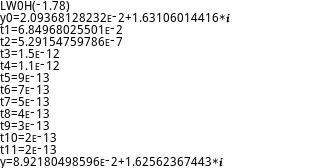11-07-2020, 07:36 AM (This post was last modified: 11-07-2020 02:41 PM by lyuka.)
Post: #13
 lyukaJunior Member Posts: 48 Joined: May 2014
RE: Lambert W function (for HP Prime)
I modified LW0H and LW0 for CAS and used them.

In CAS, both LW0 and LW0H show straightforward convergence,
and in this case shown below, correct to the last digit.
but in HOME, that is, in numerical calculation with 12 decimal digits,
convergence from around the point where the correction value reaches
several tens of ULP (unit in last place) is unnaturally slow.

In the following cases of x = -1.78, the calculation results were all
correct up to the 12th digit in CAS, but not in HOME.

LW0 Rev.1.38 convergence example at x = -1.78 at HOME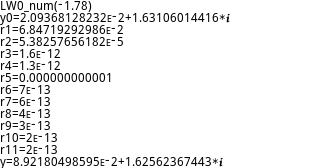LW0 Rev.1.38 convergence example at x = -1.78 in CAS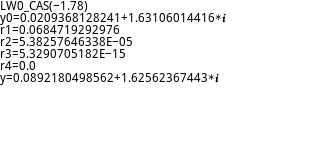LW0H Rev.1.37 convergence example at x = -1.78 at HOME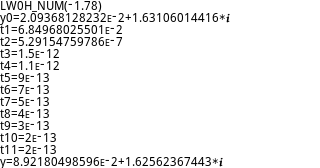LW0H Rev.1.37 convergence example at x = -1.78 in CAS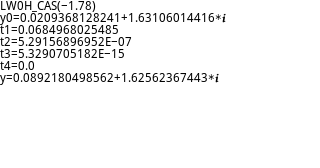Calculation result by WolframAlpha --
W0(-1.78) ≈ 0.089218049856209317716109060765294251305387407793167833503833064… + i1.6256236744277681331520891017303249073577948841857860157186500…

This convergence behavior in HOME may be due to the fact that 12 digits are not large enough, but,
it's similar to the behavior when there is a problem with normalize-and-rounding for each numerical calculation.

Here is the LW0 code for CAS used for testing.
Code:
#CAS //LW0 - Principal branch of the Lambert W function //Rev.1.38 (Nov. 7, 2020) (c) Takayuki HOSODA (aka Lyuka) LW0_CAS_test(x):= BEGIN   LOCAL y, p, s, t;    LOCAL q, r;    LOCAL n;   n:=0;   HComplex := 1; // Enable complex result from a real input.   r := 1 / e;   IF simplify(x + r) == 0 THEN return -1; END;   q := e - sqrt(2) - 1;   s := x + r;   y := ln(r + sqrt((r + r) * s) + q * s); // approximation near x=-1/e   PRINT;  // clear terminal   PRINT("LW0_CAS("+x +")");   PRINT("y" + n + "=" + y);   r := 9;   REPEAT     n := n + 1;     p := y;     q := r;     t := exp(y);     s := y * t;     t := (1 + 0.5 * y) * (s + x) + t;     IF (0 == t) THEN break; END;     y := y - (y + 1) * (s - x) / t; // Halley's method     r := abs(y - p); // correction radius;     PRINT("r" + n + "=" + r);   UNTIL 0 == r OR (q < 1 AND q <= r); // convergence check   PRINT("y=" + p);   return p; END; #END
I'm new to programming in CAS, so I'd appreciate it if you could tell me anything wrong.

Please refer to the page below for details.
Lambert W function and exponent of Lambert W function for HP Prime
11-07-2020, 01:42 PM
Post: #14
 Albert ChanSenior Member Posts: 2,357 Joined: Jul 2018
RE: Lambert W function (for HP Prime)
(11-07-2020 07:36 AM)lyuka Wrote:  Calculation result by WolframAlpha --
W0(-1.78) ≈ 0.089218049856209317716109060765294251305387407793167833503833064… + i1.6256236744277681331520891017303249073577948841857860157186500…

This convergence behavior in HOME may be due to the fact that 12 digits are not large enough, but,
it's similar to the behavior when there is a problem with normalize-and-rounding for each numerical calculation.

HOME mode imag part rounded up to is 1.62562367443, error = true - approx ≈ -0.22 ULP
However, imag part ULP is 100 times real part ULP.

HOME> y := 8.92180498562ᴇ−2+1.62562367443*i
HOME> y * e^y
−1.78-4.1692ᴇ−12*i

HOME> y := 8.92180498602ᴇ−2+1.62562367443*i
HOME> y * e^y
−1.78-1.276ᴇ−13*i

It may be why HOME mode iterations gravitate to the second number.
11-08-2020, 12:46 PM
Post: #15
 lyukaJunior Member Posts: 48 Joined: May 2014
RE: Lambert W function (for HP Prime)
(11-07-2020 01:42 PM)Albert Chan Wrote:  It may be why HOME mode iterations gravitate to the second number.

Yes, it makes sense.
I calculated and confirmed it manually using 42S.
It was really the calculation limit of a 12-digit calculator.
11-08-2020, 08:07 PM (This post was last modified: 11-11-2020 07:14 PM by lyuka.)
Post: #16
 lyukaJunior Member Posts: 48 Joined: May 2014
RE: Lambert W function (for HP Prime)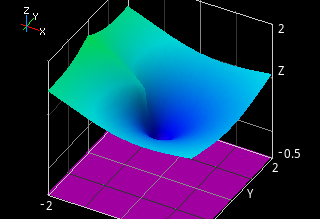Programs using the Householder's method were only a few percent faster than those using the Halley's method for their computational complexity.
It seems that the difference between cubic convergence and quaternary convergence is not so much in the calculation of about 14 decimal digits.
For now, the CAS version of the program LW0C using Halley's method seems appropriate because of its stability, accuracy, speed and simplicity.

Code:
#CAS //LW0C - Principal branch of the Lambert W function //Rev.1.40 (Nov. 12, 2020) (c) Takayuki HOSODA (aka Lyuka) //Acknowledgments: Thanks to Albert Chan for his informative suggestions. LW0C(x):= BEGIN   LOCAL y, p, s, t;   LOCAL q, r;   HComplex := 1; // Enable complex result from a real input.   r := 1 / e;   s := simplify(x + r);   IF s == 0 THEN return -1; END;   q := e - sqrt(2) - 1;   y := ln(r + sqrt((r + r) * s) + q * s); // approximation near x=-1/e   r := MAXREAL;   REPEAT     p := y;     q := r;     t := exp(y);     s := y * t;     t := (1 + 0.5 * y) * (s + x) + t;     IF (0 == t) THEN break; END;     y := y - (y + 1) * (s - x) / t; // Halley's method     r := abs(y - p); // correction radius;   UNTIL 0 == r OR q <= r; // convergence check   return p; END; #END
Lambert W function and exponent of Lambert W function for HP Prime
08-24-2022, 11:34 AM (This post was last modified: 08-25-2022 02:14 AM by Bill Triplett.)
Post: #17
 Bill TriplettJunior Member Posts: 24 Joined: Dec 2013
RE: Lambert W function (for HP Prime)
Lyuka,

Beautiful work.

When using a math function to help with designing hardware, most engineers are satisfied if the math function is accurate to six digits. Some would argue that even the first supersonic aircraft designs were accomplished using slide rules which had no better than four digits. It isn't the size of the digit count that matters. It's how you use it. The accuracy of your program is more than good enough.

I noticed my Prime generates errors if attempting to graph using the LW0H function. The LW0C function does not generate the same errors, graphs fine, and I don't see why they would be that much different.

Update:

I just noticed your discussion thread where you were battling crashes in the emulator slightly before modifying LW0C to use CAS mode. It looks as if you already tilted at that windmill. LW0C (in the current CAS form) is the victory.

I wonder if the Householder method has any advantages worth fighting the crashes.

In any case, thank you for creating each program. These are instructive.

Bill Triplett
08-25-2022, 03:17 AM (This post was last modified: 08-25-2022 12:42 PM by Bill Triplett.)
Post: #18
 Bill TriplettJunior Member Posts: 24 Joined: Dec 2013
RE: Lambert W function (for HP Prime)
When calculating values for Lambert Omega, the HP Prime can handle big numbers. For older calculators such as the HP-42S, the ancient little shirt-pocket machines were surprisingly capable.

The number of smallest subatomic particles in the observable universe is approximately 3.3x10^80. Using Lyuka's LW0C program on the Prime, we see:

LW0C(3.3E80) = 180.2

Also,

LW0C(1.797693E308) = 708.59221

This is the maximum. If we make the x input for Lyuka's LW0C program just a tiny bit larger, the result becomes undefined. If we keep perspective, and consider the size of the universe, this is not really a limit.

I polished up one of Albert Chan's Lambert Omega functions for the HP-42S. My physical machine calculates W(9.99999999999E499) = 1,144.25. No larger input is allowed.

When using the Free42 simulator, the little beast allows us to type in exponents up to 999. It can do this:

W(999,999,999,999,999E999) = 2,327.06892

We can't physically type in exponents bigger than three digits when entering a value for x, but I have an idea. Assume we have a Lambert Omega function stored in a program named "cW" in the HP-42S catalog. We can type this:

Shift
GTO
cW
999,999,999,999,999E999
ENTER
*

At this point after we hit the multiply button (*), the x register contains 1.0E2028. This is the original big number multiplied by itself.

Now, press R/S.

Mr. Chan's code calculates cW(1.0E2028) = 4661.19.

"Out of this world?" Nope. It is more like, "out of this universe."

I have no idea how far the simulated HP-42S can go.

Here is the HP-42S program I used for calculating the Lambert Omega relation values based on Mr. Chan's code:

Code:
00 { 64-Byte Prgm } 01▸LBL "cW" 02 0.3 03 -1 04 E^X 05 RCL+ ST Z 06 STO× ST Y 07 STO+ ST X 08 LASTX 09 STO+ ST Z 10 × 11 SQRT 12 + 13 X<>Y 14 +/- 15 X<>Y 16▸LBL 01 17 X=Y? 18 GTO 02 19 STO ST Y 20 LN 21 1 22 + 23 R^ 24 RCL+ ST Z 25 X<>Y 26 ÷ 27 - 28 STO× ST X 29 LASTX 30 STO+ ST Y 31 GTO 01 32 LBL 02 33 LN 34 X<>Y 35 CLX 36 STO ST Z 37 STO ST T 38 X<>Y 39 RTN 40 END
12-28-2022, 06:55 PM
Post: #19
 Albert ChanSenior Member Posts: 2,357 Joined: Jul 2018
RE: Lambert W function (for HP Prime)
(08-25-2022 03:17 AM)Bill Triplett Wrote:  Mr. Chan's code calculates cW(1.0E2028) = 4661.19.

"Out of this world?" Nope. It is more like, "out of this universe."

I have no idea how far the simulated HP-42S can go.

As high as you wanted. You can do above even on HP-12C.

a = 10^2028
x = ln(a) = 2028 * ln(10) ≈ 4669.642569      // guess for W(a)

x = ln(a) - ln(x) ≈ 4669.642569 - 8.448837810 = 4661.193731
x = ln(a) - ln(x) ≈ 4669.642569 - 8.447026860 = 4661.195542
x = ln(a) - ln(x) ≈ 4669.642569 - 8.447027248 = 4661.195542 = W(a)

With huge argument, convergence is quadratic

x * e^x = a
ln(x) + x = ln(a)

f = x + ln(x) - ln(a)
f' = 1 + 1/x

Newton's method (huge x), x = x - f/f' ≈ x - f = ln(a) - ln(x)
12-28-2022, 10:41 PM (This post was last modified: 12-28-2022 10:42 PM by toml_12953.)
Post: #20
 toml_12953Senior Member Posts: 2,046 Joined: Dec 2013
RE: Lambert W function (for HP Prime)
Here's an ANSI/ISO BASIC adaptation of a C program from

https://stackoverflow.com/questions/6021...in-c-sharp

Code:
DECLARE EXTERNAL FUNCTION LambertW PRINT LambertW(MAXNUM) END  EXTERNAL FUNCTION LambertW(x) ! LambertW is not defined in this section IF (x < EXP(-1)) THEN    PRINT "The LambertW-function is not defined for";x;"."    EXIT FUNCTION END IF ! computes the first branch for real values only ! amount of iterations (empirically found) LET amountOfIterations = MAX(4, CEIL(LOG10(x) / 3)) ! initial guess is based on 0 < ln(a) < 3 LET w = 3 * LOG(x + 1) / 4  ! Halley's method via eqn (5.9) in Corless et al (1996) FOR i = 0 TO amountOfIterations-1    LET w = w - (w * EXP(w) - x) / (EXP(w) * (w + 1) - (w + 2) * (w * EXP(w) - x) / (2 * w + 2)) NEXT i LET LambertW = w END FUNCTION

Tom L
Cui bono?
 « Next Oldest | Next Newest »

User(s) browsing this thread: 1 Guest(s)